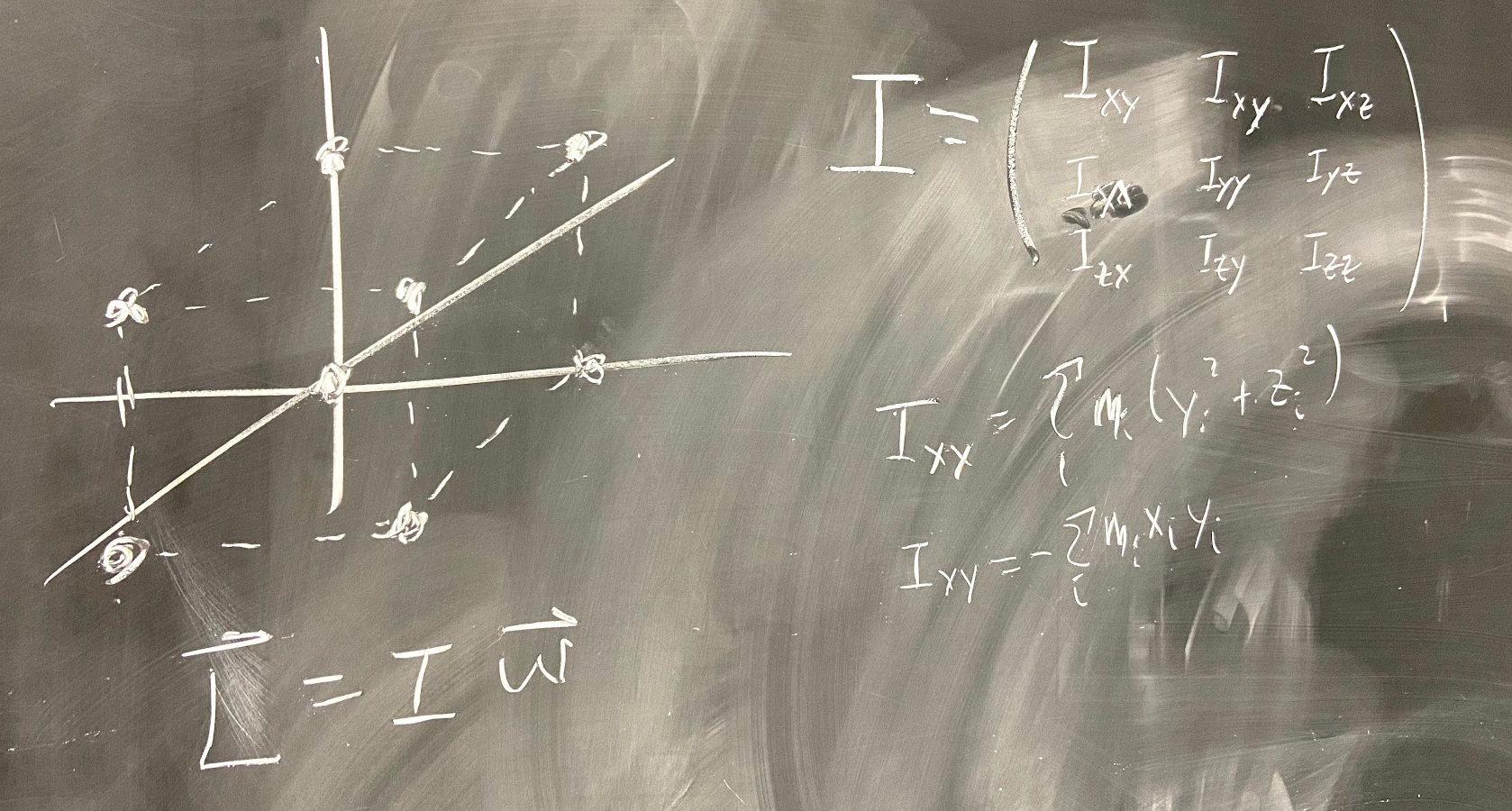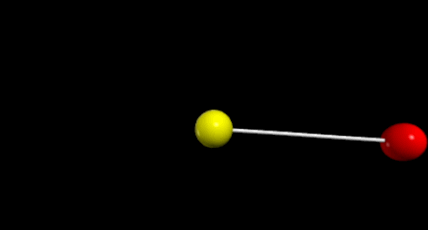# Angular Momentum and the Moment of Inertia TensorPhoto: Rhett Allain

I want to explain this cool problem, but I want to sort of give a rough outline of how to get there. It’s useful to see where things start and where they end up.

Point Masses

When you start off in your physics course, you use point masses. Oh, you don’t necessarily call them point masses — but that’s what they are. When you model a block sliding down a plane, it’s a point. When you toss a ball — yup, that’s a point.

For a point mass, we treat all the forces as acting at the same point on the object (usually the center of mass).

Two Point Masses

What’s better than one point mass? Obviously two masses are better. Let’s imagine that I have two masses connected by a spring (with no other forces acting on them).

It’s possible to model this situation by calculating the force on each mass (due to the spring) and then use the momentum principle:

Of course if both masses are moving, the vector spring force will keep changing such that’s not completely trivial to find a solution. OK, it is indeed possible if you assume an equivalent 1 body problem — here’s how to do that (but you don’t need to watch this video to follow everything here, it’s just a side quest).

But there is another way to model these two masses — a numerical calculation. If I break the motion into small intervals of time, I can approximate the spring force as a constant value during this time. I can use this constant force to find the momentum and the position at the end of the time interval. Then, I just need to repeat these calculations for the next time interval. Yes, there’s a bunch of intervals to calculate — that’s why I make a computer do it.

Here is the code for this motion. This is what it looks like.Animation: Rhett Allain

We can make it a little bit simpler if we separate this into two motions: the motion of the center of mass and the motion relative to the center of mass. Since we really just care about rotations, let’s just assume from here on that the center of mass is stationary.

There is one one way to model this motion. It’s possible to use angular momentum. For this system, we can use the angular momentum principle (along with the point particle definition of angular momentum).

Let’s just assume we have zero net torque. That means that the angular momentum must be constant. Oh, that “x” is for the cross product. Just so we are clear.

Here you can see the z-component of the total angular momentum is equal to zero (you can’t plot the full angular momentum since it’s a vector).

Nice, right? OK, there’s another way to think about this. Since these two masses are moving in a circular path, they both take the same time to complete a rotation. This means their angular velocity is also the same. If we define the angular velocity along the axis of rotation (in the +z direction using the right hand rule), then the velocity vector for each mass would be:

Here, the subscript “b” means “ball” — I just need a label. They both have the same angular velocity vector, but they will have different velocity vectors since they have different position vectors. Oh, the r-vector is from the center of mass to each ball.

Now consider the kinetic energy of this system.

Y̵e̵s̵,̵ ̵i̵f̵ ̵y̵o̵u̵ ̵t̵a̵k̵e̵ ̵t̵h̵e̵ ̵c̵r̵o̵s̵s̵ ̵p̵r̵o̵d̵u̵c̵t̵ ̵o̵f̵ ̵a̵ ̵v̵e̵c̵t̵o̵r̵ ̵w̵i̵t̵h̵ ̵i̵t̵s̵e̵l̵f̵,̵ ̵t̵h̵a̵t̵’̵s̵ ̵t̵h̵e̵ ̵s̵a̵m̵e̵ ̵a̵s̵ ̵s̵q̵u̵a̵r̵i̵n̵g̵ ̵t̵h̵e̵ ̵m̵a̵g̵n̵i̵t̵u̵d̵e̵.̵

Note: I made an error in an equation above. I have fixed it.

I’m going to skip a bunch of math, but if you replace the velocity vector with the expression for angular velocity, you can factor out the angular velocity since all the particles have this value in common. Here’s what you get.

In this expression, I is the moment of inertia. We use the same idea to get the following expression for the angular momentum.

However, we in this case, I needs to be defined as a tensor like this:

Where the double index values (xx, yy, zz) are defined as:

The off diagonal elements (xy, xz, yz…) would be:

OK, a comment. Yes, if the axis of rotation is fixed then the moment of inertia can be represented as a scalar value — but let’s stick with this definition for now.

Moment of Inertia and Angular Momentum for Two Masses

We have to do this now. Let’s find the moment of inertia tensor for the two rotating masses above. Since the elements in the tensor depend on the x,y,z values we need to know the exact position of the masses at some instant. Here is a diagram.

If the center of mass is at the origin, then the following must be true:

OK, let’s start calculating stuff. I’ll start with I_xx:

The only non-zero coordinates are in the x-direction, so this one is zero. But the other two diagonal elements have an x value in them and aren’t zero.

What about the off-diagonal elements? Since those elements are a product of two different variables, you would need both to be non-zero for the element to be non-zero. Well, that’s not going to happen here. So, here is the moment of inertia tensor.

Now let’s pick an angular velocity in the z-direction and then find the angular momentum vector. Here is my angular velocity:

Yes, I wrote this as a column vector so that I could do the multiplication with the inertia tensor.

This says that the angular momentum vector is in the same direction as the angular velocity vector — but we kind of already knew that. Still, it’s a nice place to start for a simple case.

Three Point Masses

Oh, you liked 2 masses? What about three? Suppose we have the following:

• Mass A is 0.5 kg at position <-0.05,-0.025,0> meters.
• Mass B is 1 kg at position <0.05,-0.025,0> meters.
• Mass C is 0.5 kg at position <-0.05, 0.075,0> meters

These masses are connected together by stiff springs and have an angular velocity vector of:

Here’s what that looks like. Yes, I modeled this with springs — here is the code.

OK, now for some homework.

• Find the moment of inertia tensor for these masses when they start rotating.
• Show that the angular momentum is in the same direction as the angular velocity.
• Can you find an angular velocity vector that produces an angular momentum vector in a different direction?

Note: for the above program, I picked the angular velocity vector and then calculated the initial velocity vector for each mass using the following:

Rigid Body With 4 Masses

Why not move up to 4 masses? Let’s do it. I’ll start with my numerical calculation using springs to connect all the masses in a rigid-like manner. Here is the code followed by an animation.

Clearly, this is going to get more complicated. But here’s what I want to do:

• Find the moment of inertia tensor at t = 0 seconds.
• Start with an initial angular velocity vector and find the angular momentum vector.
• Show that angular momentum is constant.
• Let it rotate a bit and then find the moment of inertia tensor again — see if the same angular velocity vector gives the same angular momentum vector.

Let’s get started. Here is a starter program with just the masses in their initial configuration (that why I don’t have to write out the values).

I probably should have made these 4 masses as a list — oh well, I will do that later. The next thing I’m going to do is to build a function to create the moment of inertia tensor. Since GlowScript doesn’t have a built in class for tensors or matrices, I’m going to make my own. The tensor is 3 x 3, so I will just make it a list of three vectors.

Wait. What the heck is a list? Here is my short intro:

So, this is what an inertia tensor would look like in python.

Cool? OK, let’s make a function that takes the four masses and their vector locations and then returns a moment of inertia tensor. Oh, what the heck — I’m going to include a point about which the tensor is calculated (it doesn’t actually have to be the origin).

Check it out. Here is my function (I added it to the end of that same program above).

Some comments on this code:

• Line 58: Yes, I made this for explicitly 4 masses. I could have made a generic function for any number of masses — I will assign that as homework to myself.
• Line 61: Just to make things easier, I added the four masses to a list.
• Line 62–67: Remember each element in the tensor is a sum over all the masses. Since it’s s a sum, it has to start at 0.
• Line 68–78: Here’s the key calculation. Remember, I don’t need to calculate both Ixy AND Iyx — they are the same.

When I run this, I get the following inertia tensor:

Seems legit. Next step is to build a function that operates a tensor and a vector. It’s essentially a bunch of dot products. Maybe this diagram showing a 2x2 and a 2x1 vector will help.

The result of this will be a 2x1 vector. The first element in the resultant will be the dot product between the two red arrows. The second element will be the dot product between the blue arrows.

Now I just need to build this function — there’s already a function for the dot product, so I can use that.

I think that one is straight forward — no need for comment.

OK, I’m going to start with an angular velocity of <0,0,4> rad/s. With that, I get the following angular momentum:

Now, I need to let it rotate for a short bit and then find the new moment of inertia tensor. I know the angular momentum is constant (using r x p for each mass from before). Notice that I can use the angular velocity vector to find the velocities of each particle — however, I can’t go backwards. You can’t use the velocity of the particles to find the angular velocity. That’s why I’m doing this weird method.

After a rotation, I get the following inertia tensor and calculated angular momentum (based on an angular velocity of <0,0,4> rad/s.

But what does it all mean? This means that:

• Yes, the moment of inertia tensor changes as the object rotates.
• Using the same angular velocity as before, I get a different angular momentum.
• Since I know the angular momentum is actually constant, that means that’s not the actual angular velocity. The angular momentum and the angular velocity are in different directions — and that’s OK.

So, what’s next? Well, it just so happens that there are some directions for the angular velocity vector such that the angular momentum is in the same direction. Yes, that will be a future giant post.

Physics faculty, science blogger of all things geek. Technical Consultant for CBS MacGyver and MythBusters. Former WIRED blogger.

Physics faculty, science blogger of all things geek. Technical Consultant for CBS MacGyver and MythBusters. Former WIRED blogger.